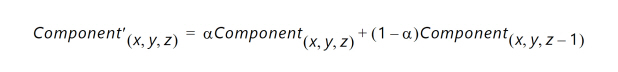# Alpha Blending - 5.2 English

## PG243 Video Mixer

Document ID
PG243
Release Date
2022-10-19
Version
5.2 English

Alpha blending is the process of combining two images with the appearance of partial transparency. To perform this composition, a per layer alpha value (and optionally a per pixel alpha value) is used that contains the coverage information for all the pixels within a layer. The alpha value ranges from 0 to 1, where 0 represents that the current pixel does not contribute to the final image and is fully transparent. A 1 represents that the current pixel is fully opaque. Any value in between represents a partially transparent pixel.

When applying alpha blending, the two pixels to be blended reside within two different image layers. Each layer has a definite Z-axis order. In other words, each layer resides closer or farther from the observer and has a different depth. Thus, the image pixel and the image pixel directly "over" it are to be blended.

The equation for alpha blending one layer to the layer directly behind in the Z-axis is below. This operation is conceptually simple linear interpolation between each color component of each layer.Where:

a is the product of the global alpha and per pixel alpha (if enabled) or just the global alpha value (otherwise).

Component (x, y, z) represents one color component channel from the color space triplet (RGB, YUV, etc.) associated with the pixel at coordinates ( x, y ) in Layer z.

Component (x, y, z – 1) represents the same color component at the same ( x, y ) coordinates in Layer z – 1 (one layer below in Z-plane order).

Component' (x, y, z) is the resulting output component value after alpha-blending the component values from coordinates ( x, y ) from Layer z and Layer z – 1.

The same equation applies for the next layer above, Layer z + 1. These alpha-blending operations can be chained together by taking the resultant output, Component' (x, y, z) , and substituting it into the Layer z + 1 equation for Component (x, y, z) . This implies that the result of blending Layer z with the background becomes the new background for Layer z + 1, or the layer directly over it. In this mode, any number of image layers can be blended by taking the blended result of the layer below it.

Note: Alpha blending is optional per layer but always enabled for the logo layer. In case alpha blending is disabled, pixels are always superimposed as fully opaque on the underlying layer.